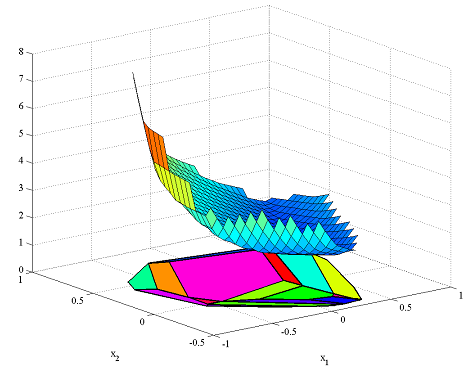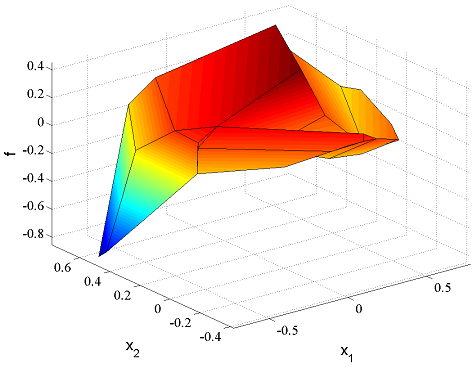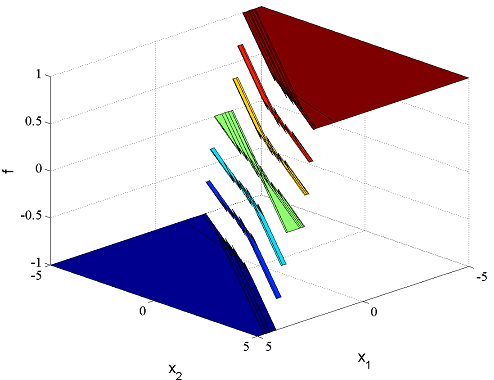This tutorial requires MPT.

YALMIP can be used to calculate explicit solutions of parametric linear and quadratic programs by interfacing the Multi-Parametric Toolbox MPT. This tutorial assumes that the reader is familiar with parametric programming and the basics of MPT.

### Generic example.

Consider the following simple quadratic program in the decision variable z, solved for a particular value on a parameter x.

A = randn(15,3);
b = rand(15,1);
E = randn(15,2);

z = sdpvar(3,1);
x = [0.1;0.2];

F = [A*z <= b+E*x];
obj = (z-1)'*(z-1);

sol = optimize(F,obj);
value(z)
ans =
-0.1454
-0.1789
-0.0388


To obtain the parametric solution with respect to x, we call the function solvemp, and tell the solver that x is a parametric variable. Moreover, we must add constraints on x to define the region where we want to compute the parametric solution, the so called exploration set.

x = sdpvar(2,1);
F = [A*z <= b+E*x, -1 <= x <= 1];
sol = solvemp(F,obj,[],x);


The first output is an MPT structure. In accordance with MPT syntax, the optimizer for the parametric value (0.1,0.2) is given by the following code.

xx = [0.1;0.2];
[i,j] = isinside(sol{1}.Pn,xx)
sol{1}.Fi{j}*xx + sol{1}.Gi{j}
ans =
-0.1454
-0.1789
-0.0388


By using more outputs from solvemp, it is possible to simplify things considerably.

[sol,diagnostics,aux,Valuefunction,Optimal_z] = solvemp(F,obj,[],x);


The function now returns solutions using YALMIPs nonlinear operator framework. To retrieve the numerical solution for a particular parameter value, simply use assign and value in standard fashion.

assign(x,[0.1;0.2]);
value(Optimal_z)


Some of the plotting capabilities of MPT are overloaded for the piecewise functions. Hence, we can plot the piecewise quadratic value function

plot(Valuefunction);
figure
plot(Optimizer);and plot the piecewise affine optimizer

figure
plot(Optimizer(1));### Simple MPC example

Define numerical data for a linear system, prediction matrices, and variables for current state $$x$$ and the future control sequence $$U(x)$$, for an MPC problem with horizon 5 (create_CHS is a cheat function that creates the numerical matrices to describe the linear relation between current state $$x$$ and future input sequence $$U$$, to the predicted outputs. See the standard MPC example to see how you would do this in a more generic fashion in an actual application)

N = 5;
A = [2 -1;1 0];
B = [1;0];
C = [0.5 0.5];
[H,S] = create_CHS(A,B,C,N);
x = sdpvar(2,1);
U = sdpvar(N,1);


The future output predictions are linear in the current state and the control sequence.

Y = H*x+S*U;


We wish to minimize a quadratic cost, compromising between small input and outputs.

objective = Y'*Y+U'*U;


The input variable has a hard constraint, and so does the output at the terminal state.

F = [1 >= U >= -1, 1 >= Y(N) >= -1];


We seek the explicit solution $$U(x)$$ over the exploration set $$\left \lvert x\right \rvert \leq 5$$

F = [F, 5 >= x >= -5];


The explicit solution $$U(x)$$ is obtained by calling solvemp with the parametric variable $$x$$ as the fourth argument. Additionally, since we only are interested in the first element of the solution $$U(x)$$, we use a fifth input to communicate this.

[sol,diagnostics,aux,Valuefunction,Optimizer] = solvemp(F,objective,[],x,U(1));


We can plot the overloaded solutions directly

figure
plot(Valuefunction)
figure
plot(Optimizer)


### Mixed integer multiparametric programming

YALMIP extends the multiparametric solvers in MPT by adding support for binary variables in the parametric problems.

Let us solve an extension of the MPC problem from the previous section. To begin with, we formulate a similar problem (shorter horizon and linear cost)

N = 3;
A = [2 -1;1 0];
B = [1;0];
C = [0.5 0.5];
[H,S] = create_CHS(A,B,C,N);
x = sdpvar(2,1);
U = sdpvar(N,1);
Y = H*x+S*U;

objective = norm(Y,1) + norm(U,1);

F = [1 >= U >= -1];
F = [F, 5 >= x >= -5];


We will now solve this problem under the additional constraints that the input is quantized in steps of 1/3. This can easily be modelled in YALMIP using ismember. Note that this nonconvex operator introduces a lot of binary variables, and the MPC problem is most likely solved more efficiently using a dynamic programming approach.

F = [F, ismember(U,[-1:1/3:1])];


Same commands as before to solve the problem and plot the optimal solution

[sol,diagnostics,aux,Valuefunction,Optimizer] = solvemp(F,objective,[],x,U(1));
plot(Optimizer);For more examples, see the dynamic programming example, the robust MPC example, the portfolio example, and the MAXPLUS control example.